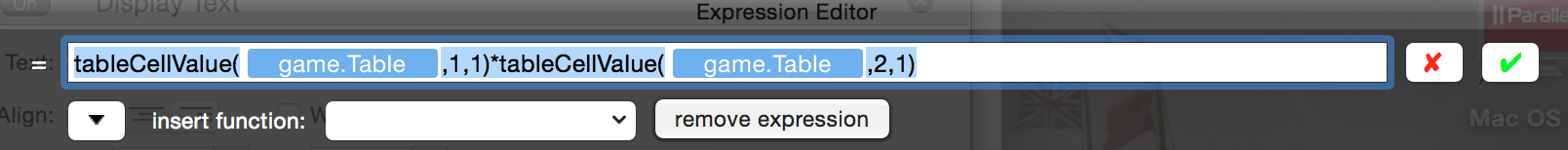# Multiplication or Table Values

Hi Guys,

I was hoping someone could tell me how I can multiply numbers within a table.
For example, if I had "2" in column 1 row 1 and then "3" in column 1 row 2 then "4" in column 1 row 3....how can I multiply these numbers to give me "24" (i.e. 2x3x4)??
Is this possible??

I really look forward to your response.

• edited August 2014

Yes, this would be possible. You´ll need a attribute to collect the value in, so create a integer and call it multiply or something
And then when you want to calculate the answer just do
Change attribute game.multiply to ("In your case")
tableCellValue(game."YourTable",1,1)tableCellValue(game."YourTable",2,1)tableCellValue(game."YourTable",3,1)

Edit:
Here is an example
https://www.dropbox.com/s/91ska8m7xxq6tbl/Multiply.zip

• Thanks Fajlajp, I tried this but I placed 'x' (multiplication symbol) between each table cell value which obviously caused my issue.
Is there a maximum amount of table cell value multiplication processes that can be placed in an equation? (I.e. Could I multiply 100 numbers this way or would there be an easier way?

• edited August 2014

You don't need any extra attribute (but you might - depends on what you're going to do with the result), but you do need to use * to multiply, not x.

For example:I had this function just in a Display Text behaviour.

As for your other question, yes you can do that with a loop, like this:

--Loop when x <= tableRowCount(yourtable)
----Change Attribute game.multiply to game.multiply * tableCellValue(yourtable, x, 1)
----Change Attribute x to x + 1

x is just a dummy attribute that's only used for the loop, so it is preferely a self attribute. I took for granted that you wanted to multiply all rows, but if you want to change that, replace the tableRowCount(yourtable) with a number or an attribute telling how many rows. The multiply attribute needs to be 1 as default, or this will not work.

Windows users don't have loops yet, but it's possible to do a work-around, just search how to do it.

I hope this will work for you and good luck in the future!• Thanks everyone for your responses. I actually did use "*" not "x" originally but it didn't seem to work.
I will try this again and let you know if there are any issues.